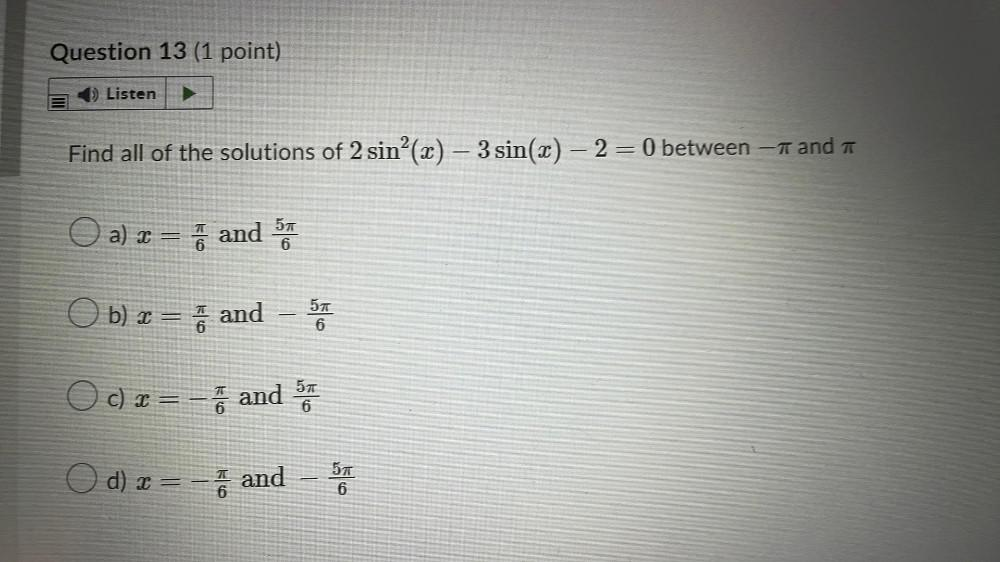Question:

# Find all of the solutions of 2 sin²(x) - 3 sin(x) - 2 = 0

Last updated: 7/31/2022Find all of the solutions of 2 sin²(x) - 3 sin(x) - 2 = 0 between - π and π a) x = π/6 and 5π/6 b) x = π/6 and -5π/6 c) x =-π/6 and 5π/6 d) x =− π/6 and – 5π/6